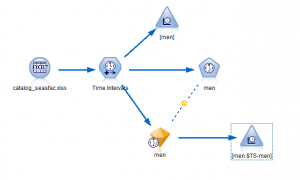# SPSS Modeler下sav格式转成csv格式

13537℃

```#coding: utf-8
import os
import glob
import numpy as np
import pandas as pd

'''
.sav - the IBM SPSS Statistics Input Output Module
'''

def convertSavToCsv(srcFile):
fpathandname, fext = os.path.splitext(srcFile)
assert fext == '.sav'
desFile = fpathandname+'.csv'
names = fp.varNames
types = fp.varTypes
# for line in fp:
#     print line
records = fp.all()
# print records
# records_array = np.array(records)
# print records_array
records_dataframe = pd.DataFrame(records, index=None, columns=names)
# print records_dataframe
records_dataframe.to_csv(desFile, index=0)

if __name__ == '__main__':
filename = 'someFile.sav'
convertSavToCsv(filename)
for filename in glob.glob('./*.sav'):
print filename
convertSavToCsv(filename)
```